## Fast Convolution and Multiplication

Algorithm 2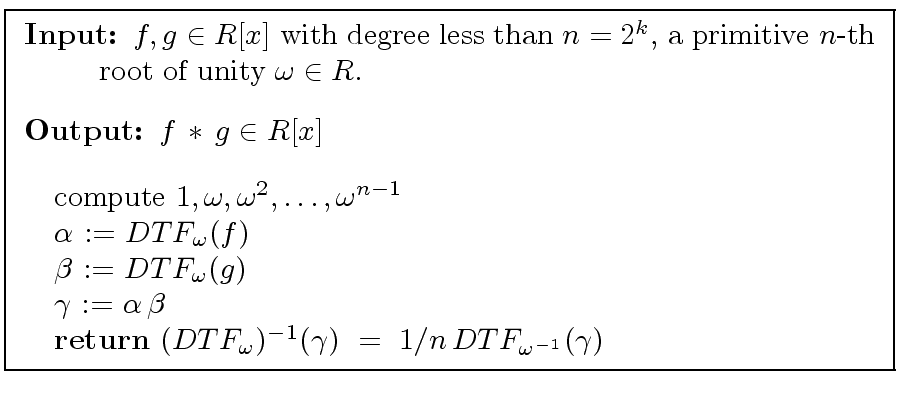Theorem 2   Let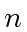be a power of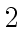and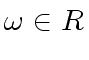a primitive-th root of unity. Then convolution in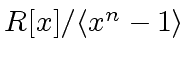and multiplication in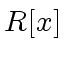of polynomials whose product has degree less thancan be performed using
•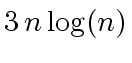additions in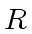,
•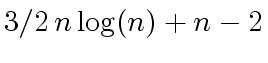multiplications by a power of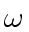,
•multiplications in,
•divisions by(as an element of),
leading to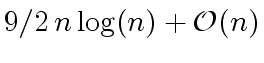operations in.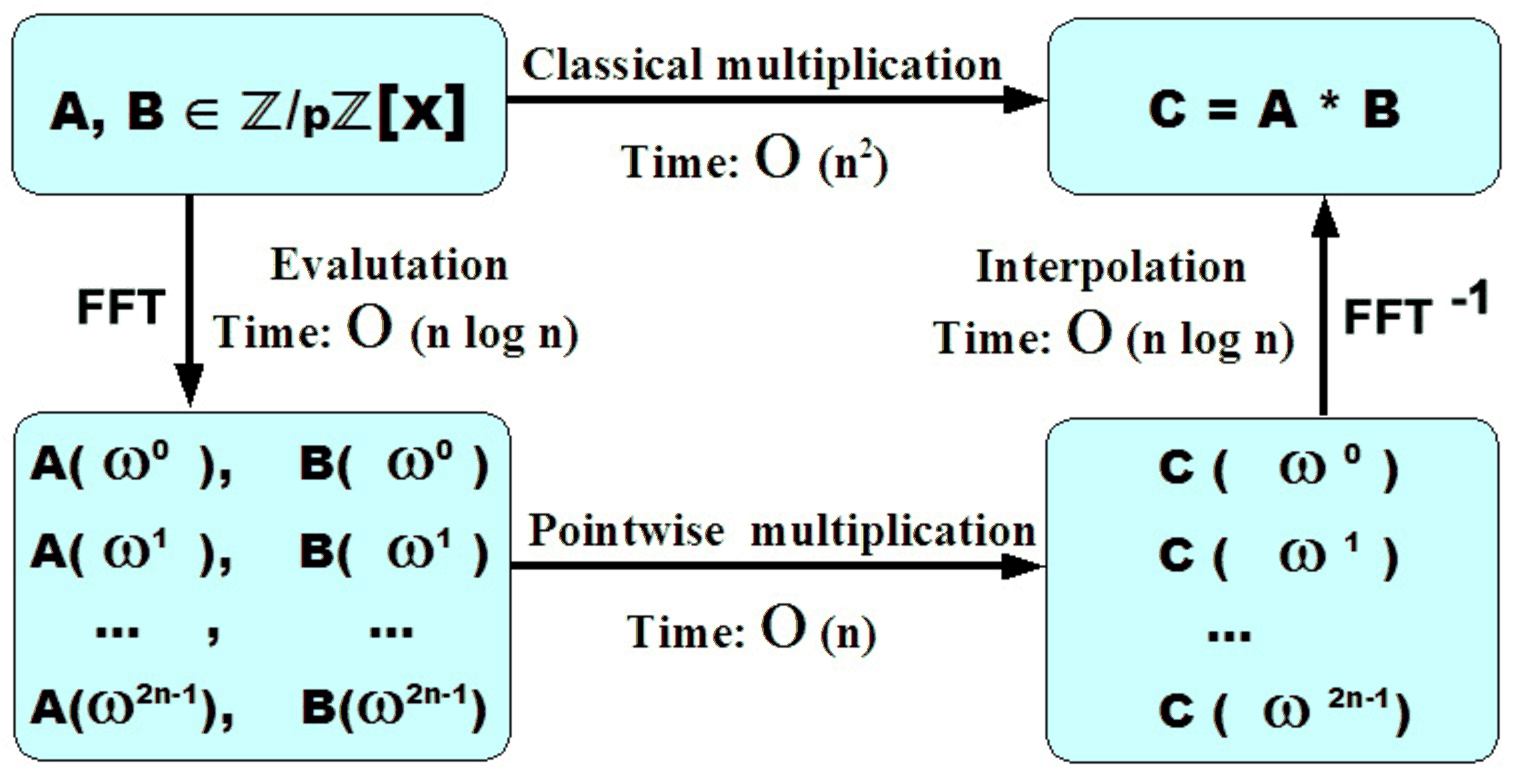Remark 4   To multiply two arbitrary polynomials of degree less than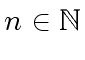we only need a primitive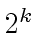-th root of unity where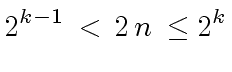(41)

Then we have decreased the cost of about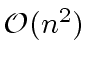of the classical algorithm to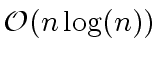.

Marc Moreno Maza
2008-01-07